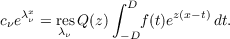#### Vol. 16, No. 1, 1966

 Download this articleFor screen For printingRecent Issues Vol. 325: 1 Vol. 324: 1  2 Vol. 323: 1  2 Vol. 322: 1  2 Vol. 321: 1  2 Vol. 320: 1  2 Vol. 319: 1  2 Vol. 318: 1  2Online Archive Volume: Issue:The Journal Subscriptions Editorial Board Officers Contacts Submission Guidelines Submission Form Policies for Authors ISSN: 1945-5844 (e-only) ISSN: 0030-8730 (print) Special Issues Author Index To Appear Other MSP Journals
On the stability of the set of exponents of a Cauchy exponential series

### S. Verblunsky

Vol. 16 (1966), No. 1, 175–188
##### Abstract

If f L(D,D) and Q(z) is a meromorphic function whose poles, all simple, forms a sub-set of the set {λν}(ν = 0,±1,±2,), then the C.E.S. (Cauchy exponential series) of f with respect to Q(z) is cνeλνx, whereSuppose we are given a class A of functions f each of which can be ‘represented’ in (D,D) by its C.E.S. with respect to Q(z). We define a set of neighbourhoods U of {λν}. Then {λν} is stable if there is a U such that to each {κν}∈ U there corresponds a meromorphic function q(z) whose poles, all simple, form a sub-set of {κν} and which is such that each f A can be represented in (D,D) by its C.E.S. with respect to q(z); and {λν} is unstable if there is no such neighbourhood.

The case in which λν = , A is BV [D,D], ‘representation of f in (D,D)’ means ‘ |ν|ncνeλνx 12(f(x+) + f(x)) boundedly within (D,D)’ is considered. It is shown, in particular, that with reasonable conditions on the set of neighbourhoods U, {} is unstable if D > 12π, and stable if D = 12π.

Primary: 30.60
##### Milestones